## Normal Vector

The normal to a Plane specified by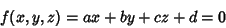(1)

is given by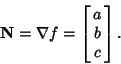(2)

The normal vector at a point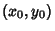on a surface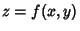is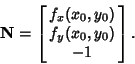(3)

In the Plane, the unit normal vector is defined by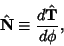(4)

where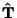is the unit Tangent Vector and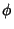is the polar angle. Given a unit Tangent Vector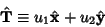(5)

with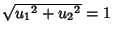, the normal is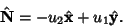(6)

For a function given parametrically by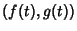, the normal vector relative to the pointis therefore given by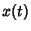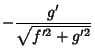(7)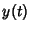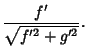(8)

To actually place the vector normal to the curve, it must be displaced by.

In 3-D Space, the unit normal is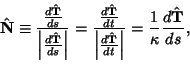(9)

where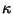is the Curvature. Given a 3-D surface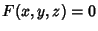,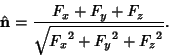(10)

If the surface is defined parametrically in the form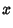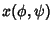(11)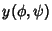(12)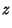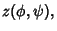(13)

define the Vectors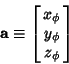(14)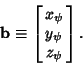(15)

Then the unit normal vector is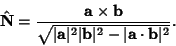(16)

Let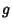be the discriminant of the Metric Tensor. Then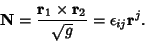(17)

Gray, A. Tangent and Normal Lines to Plane Curves.'' §5.5 in Modern Differential Geometry of Curves and Surfaces. Boca Raton, FL: CRC Press, pp. 85-90, 1993.